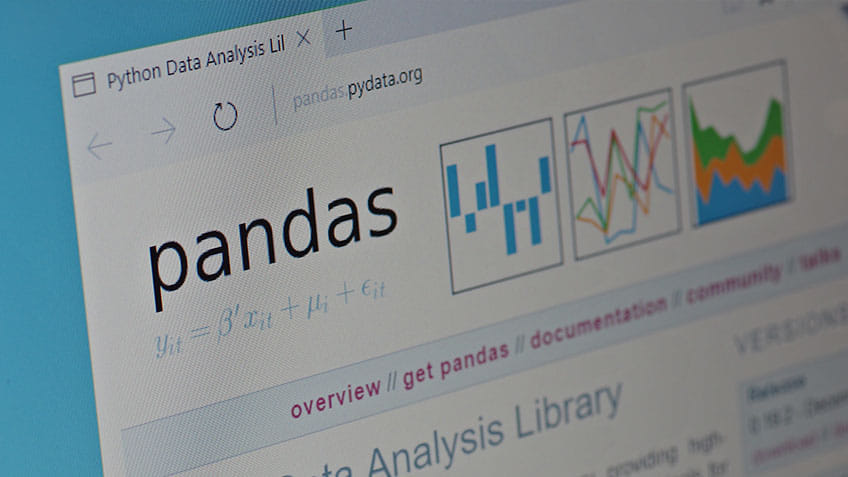### Tutorial PlaylistPython pandas is one of the most widely-used Python libraries in data science and analytics. It provides high-performance, easy-to-use structures, and data analysis tools. Two-dimensional table objects in pandas are referred to as DataFrame, as well as Series. It is a structure that contains column names and row labels.

• What are Python pandas?
• Pandas Series
• Basic Operations on Series
• Pandas DataFrames
• Basic Operations on DataFrames
• Python Pandas: Sorting
• Python Pandas: Merging
• Python Pandas: GroupBy
• Python Pandas: Concatenation

## What is Python Pandas?

Pandas is an open-source Python library that provides high-performance, easy-to-use data structure, and data analysis tools for the Python programming language

Python with pandas is used in a wide range of fields, including academics, retail, finance, economics, statistics, analytics, and many others.

Python pandas is well suited for different kinds of data, such as:

• Ordered and unordered time series data
• Unlabeled data
• Any other form of observational or statistical data sets

#### Python Training Course

Learn Data Operations in Python## Pandas Series

Series is a one-dimensional array that can contain any type of data. You can create a series by using the following constructor:

pandas.Series(data, index, dtype, copy)

Example:Fig: importing pandas module

## Basic Operations on Series

• Create a series from ndarrayFig: ndarray series

If you don’t mention the index of the array, it begins at zero by default.

• Create a series from a dictionary

A dictionary data structure can be passed as an input in the series.

Example:Fig: Series from a dictionary

• Accessing data from a series

To access the data in the series, we enter the index number of the element or the label on an element.

Example:Fig: Access data in a series

To retrieve data using labels, we enter the label value.

Example:Fig: Retrieving data by label name

## Pandas DataFrame

A DataFrame is a multi-dimensional data structure in which data is arranged in the form of rows and columns. You can create a DataFrame using the following constructor:

pandas.DataFrame(data, index, columns, dtype, copy)

Example:Fig: Empty DataFrame

## Basic Operations on DataFrames

• Create a DataFrame from lists

A DataFrame can be created using a list:Fig: DataFrameFig: 2-D DataFrame

• Creating a DataFrame from a series dictionary

A series dictionary can be passed to form a DataFrame.

Example:Fig: DataFrame from a Series dictionary

Let us now look at the column selection, addition, and deletion, and indexing a DataFrame through an example.

• Column selection

You select a particular column by mentioning the column name.

Example:Fig: Column selection

• Addition of a new column

The following enables users to incorporate new columns into the data provided:• Deleting a column

Columns can be deleted using the del or pop functions.

Example:Fig: Deleting a column

• Indexing a DataFrame

The iloc() method is used for integer-based indexing.

Example:Fig: iloc()

## Python Pandas Sorting

There are two types of sorting available in pandas. They are:

• By label
• By actual value

• ### By Label

The sort_index() method is used to sort data in pandas. You pass the axis arguments and order of the sorting.

Example:Fig: Sorting by label

By default, sorting is done in ascending order.

• ### By Actual Value

The sort_values() method is used to sort the column according to values.

Example:Fig: By actual value

## Python Pandas GroupBy

The groupby function performs one of the following operations on original data. They include:

• Splitting the object
• Applying a function
• Combining the result

Let’s create a DataFrame object and perform all the operations.

Example:Fig: DataFrame

### Split Data by Groups

Let us see how grouping objects can be used in DataFrames.

Example:Fig: Splitting data into groups

### View GroupsFig: View groups

## Python Pandas: Merging

You can merge two DataFrames by including the key in the following way:Fig: Merging two DataFrames

In the above program, we used the ‘id’ column as a common key.

## Python Pandas: Concatenation

The concat function is used to concatenate two DataFrames.

Example:Fig: Concatenation

Looking forward to make a move to programming? Take up the Python Training Course and begin your career as a professional Python programmer.

## Conclusion

In this Python pandas tutorial, we covered Python pandas and its different functions. We also provided a visual example that demonstrated how to use DataFrames and Series in Python pandas.

If you have any questions or comments, please post them below, and we'll have our experts get back to you as soon as possible.Simplilearn